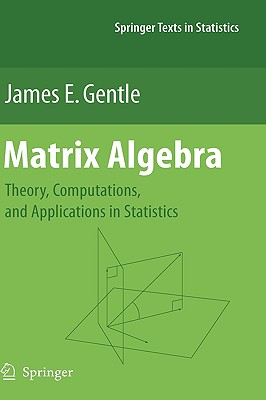### Matrix Algebra (Hardcover)

Theory, Computations, and Applications in Statistics (Springer Texts in Statistics)

Springer, 9780387708720, 528pp.

Publication Date: July 27, 2007

Other Editions of This Title:
Paperback (11/19/2010)

List Price: 109.99*
* Individual store prices may vary.

#### Description

Matrix algebra is one of the most important areas of mathematics for data analysis and for statistical theory. The first part of this book presents the relevant aspects of the theory of matrix algebra for applications in statistics. This part begins with the fundamental concepts of vectors and vector spaces, next covers the basic algebraic properties of matrices, then describes the analytic properties of vectors and matrices in the multivariate calculus, and finally discusses operations on matrices in solutions of linear systems and in eigenanalysis. This part is essentially self-contained.

The second part of the book begins with a consideration of various types of matrices encountered in statistics, such as projection matrices and positive definite matrices, and describes the special properties of those matrices. The second part also describes some of the many applications of matrix theory in statistics, including linear models, multivariate analysis, and stochastic processes. The brief coverage in this part illustrates the matrix theory developed in the first part of the book. The first two parts of the book can be used as the text for a course in matrix algebra for statistics students, or as a supplementary text for various courses in linear models or multivariate statistics.

The third part of this book covers numerical linear algebra. It begins with a discussion of the basics of numerical computations, and then describes accurate and efficient algorithms for factoring matrices, solving linear systems of equations, and extracting eigenvalues and eigenvectors. Although the book is not tied to any particular software system, it describes and gives examples of the use of modern computer software for numerical linear algebra. This part is essentially self-contained, although it assumes some ability to program in Fortran or C and/or the ability to use R/S-Plus or Matlab. This part of the book can be used as the text for a course in statistical computing, or as a supplementary text for various courses that emphasize computations.

The book includes a large number of exercises with some solutions provided in an appendix.